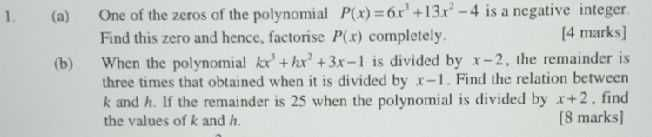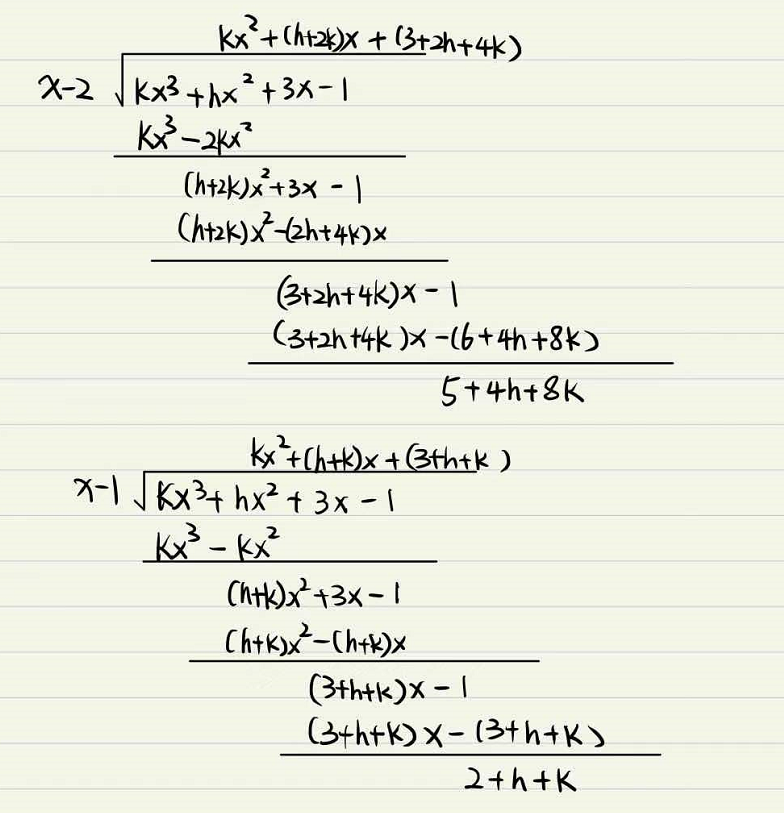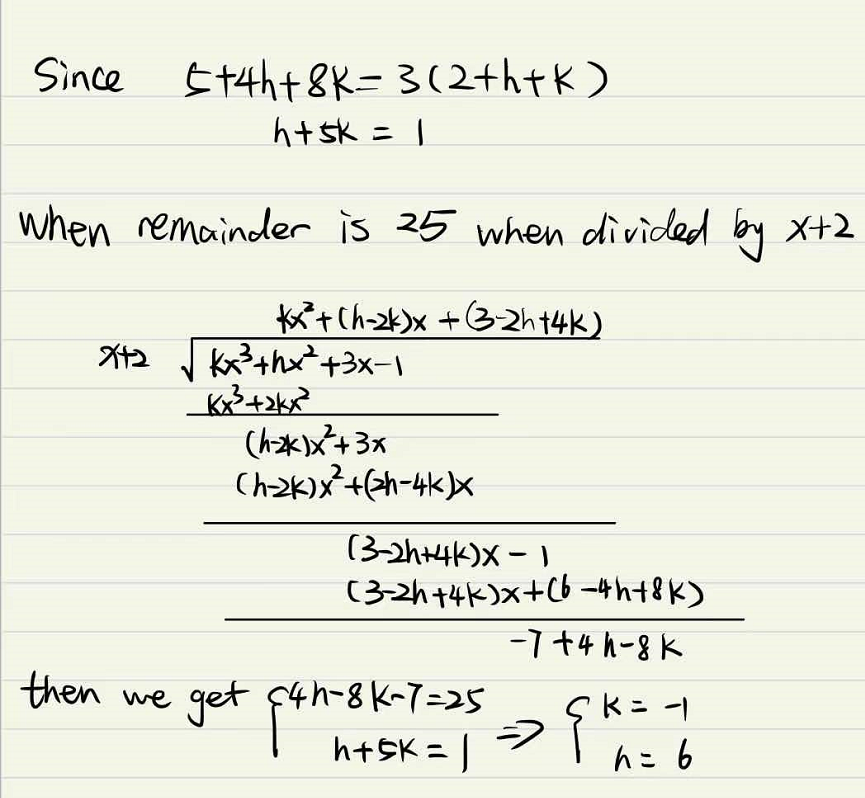### ¿Todavía tienes preguntas de matemáticas?

Pregunte a nuestros tutores expertos
Algebra
Pregunta1. (a) One of the zeros of the polynomial $$P ( x ) = 6 x ^ { 3 } + 13 x ^ { 2 } - 4$$ is a ncgative integer. Find this zero and hence, factorise $$P ( x )$$ completely.

(b) When the polynomial $$k x ^ { \prime } + h x ^ { 2 } + 3 x - 1$$ is divided by $$x - 2$$ , Ihe remainder is three times that obtaned when it is divided by $$x - 1$$ . Find the relation between $$k$$ and $$h$$ . If the remainder is $$25$$ when the polynomial is divided by $$x + 2$$ . find the values of $$k$$ and $$h$$ .

$$[ 8$$ marks]